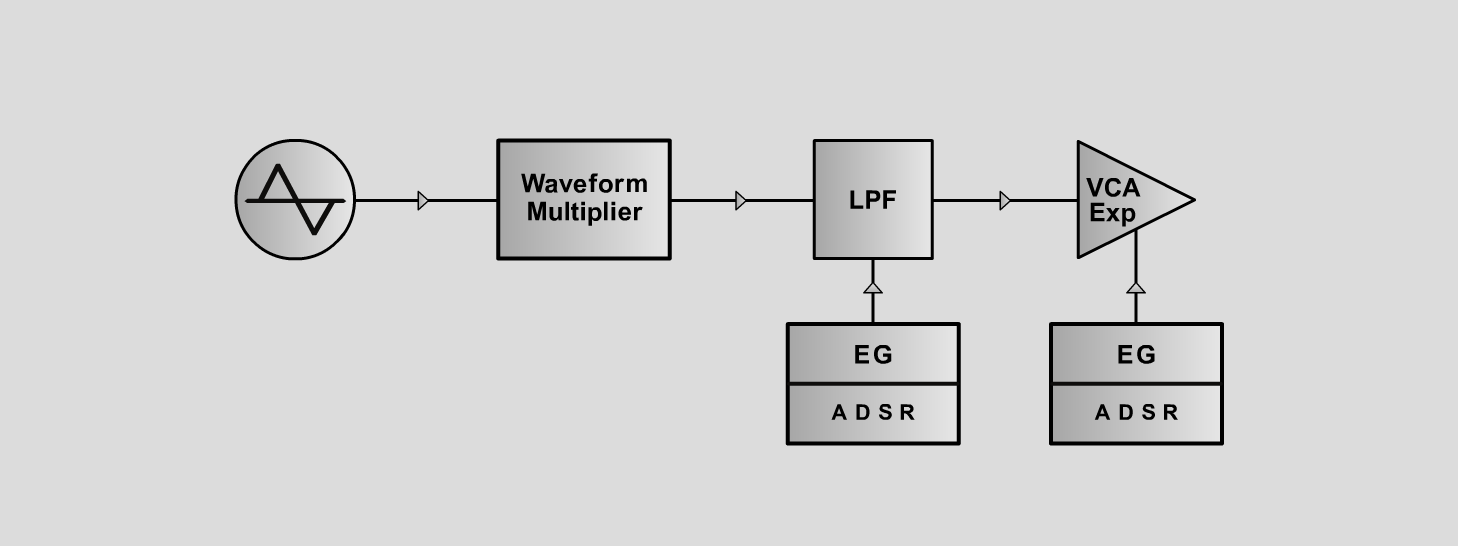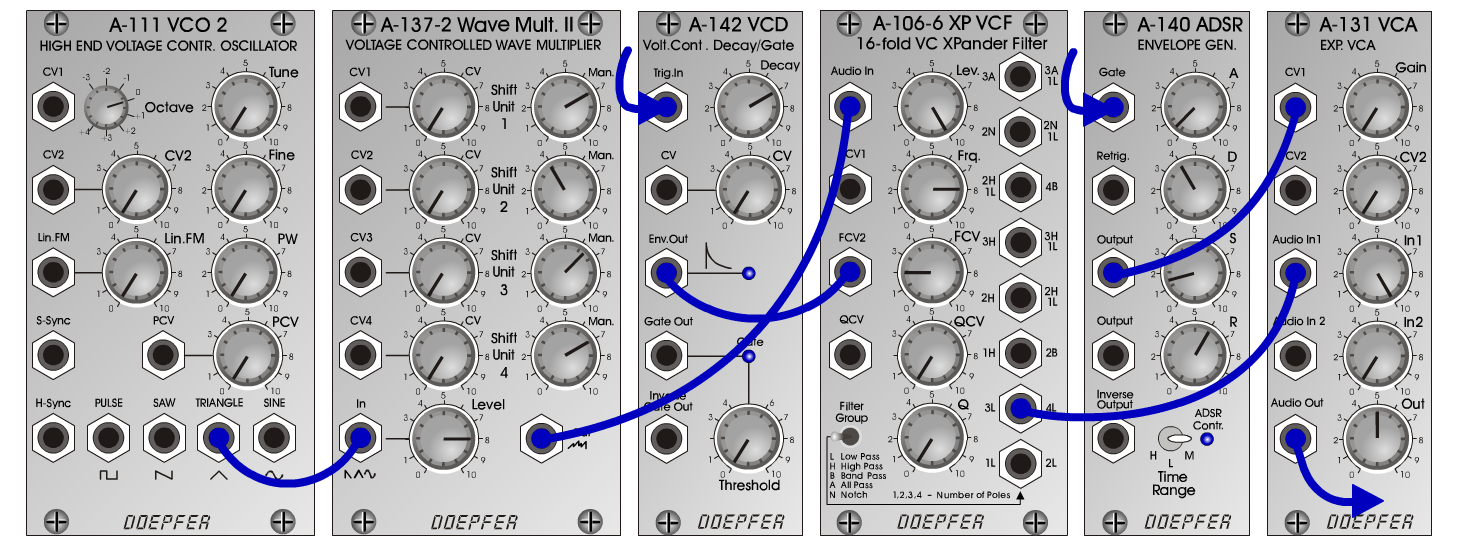MandolinInstrumentsD o e p f e r A - 100 Connections: Settings: A-111 (Tri) <=> A-137-2 (In) A-137-2 (Out) <=> A-106-6 (Audio In) A-106-6 (3L) <=> A-131 (Audio In) A-140 (Output) <=> A-131 (CV1) A-142 (Env Out) <=> A-106-6 (FCV2) A-137-2 (Man 1 = 7, Man 2 = 4, Man 3 = 6.5, Man 4 = 7, Level = 8) A-106-6 (Lev = 10, Frq = 8, FCV = 2, QCV = 0, Q =0) A-140 (A = 0.5, D = 4, S = 1.5, R = 6, Range = M) A-131 (Gain = 0, Audio In 1 = 10, Audio Out = 5) MandolinL:  A-140 (A = 0.5, D = 4, S = 5, R = 6, Range = M) Josef MuellerSound samples Vivaldi: Concerto per Mandolino (RV 425) Mandolin (523 Hz) Mandolin (1046 Hz)